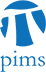## Topology Seminar: An infinite loop space machine for symmetric monoidal 2-categories

• Date: 01/20/2010
Lecturer(s):
Angelica Osorno (MIT)
Location:

University of British Columbia

Description:

In recent work of Baas-Dundas-Richter-Rognes, the authors prove that
the classifying space of 2-vector bundles, K(Vect) is equivalent to the
algebraic K-theory of the connective K-theory spectrum ku. In this talk
we will show that K(Vect) is the group completion of the classifying
space of the 2-category of 2-vector spaces, which is a symmetric
monoidal 2-category. We will explain how to use the symmetric monoidal
structure to produce a $\Gamma$-2-category, which will give an infinite
loop space structure on K(Vect). Then we will show that the equivalence
of BDRR is a map of infinite loop spaces.

Schedule:

3:00pm - 4:00pm, WMAX 110